# A Sunday excursion in integral land

$\int \frac{1}{e^x + 1}\mathrm{d} x$

Those who do not immediately recognise the technique as described below may try the substitution $u = e^x+1$ which converts the integral into

$\int \frac{\mathrm{d} u}{u\left( u-1 \right)}$

which calls for the treatment of partial fractions, but such is unnecessary.

There exists a very simple solution to this integral, by means of converting the numerator to $e^x$ via the relation

$\int \frac{1}{e^x + 1}\mathrm{d} x\ = \int \left( 1 - \frac{e^x}{e^x + 1} \right)\mathrm{d} x$

from which we can solve for the integral. But when I revisited this integral earlier today, I wanted to try something new.

Instead of converting the original integral with a denominator of $e^x+1$ to a rational function in the first method, I wanted to try to convert a rational function to another that involved $e^x$.

Essentially, this could be done with $\int \frac{\mathrm{d} x}{x\left( x-1 \right)}$ and the deliberate substitution $x=e^u+1$, which basically yields the same answer as if you did the integral with partial fractions (which are very easily found), except you purposefully avoided using them for reasons unknown.

I wanted to take this one step further. I went ahead and tried doing this same with a generalised form of a rational function that takes the form of a fraction with a quadratic as the denominator and a constant for the numerator.

$\int\frac{\mathrm{d} x}{x^2+ax+b}$

Note that if the numerator had been a linear function, the integral can first partially be solved by scaling the numerator to be of the form $2x+c$, from which it is apparent that $ln|x^2+ax+b|$ forms part of the solution. If the numerator has a rational function of powers higher, simply dividing the excess off the numerator would reduce the integral to the above cases.

The numerator is set to be just 1, since I could just scale it with a constant at any time, and the quadratic is reduced to have its leading coefficient to be again just 1.

Referring to what I did for the integral that could have been done with partial fractions instead, I first factored the denominator, then tried the same trick, deliberately including the substitution of $x=e^u+c$ and $dx=e^u\mathrm{d} u$. I saw that the $e^u$ would cancel, leaving me with an integral similar to that of the first integral on this page, which sparked my curiosity for this problem.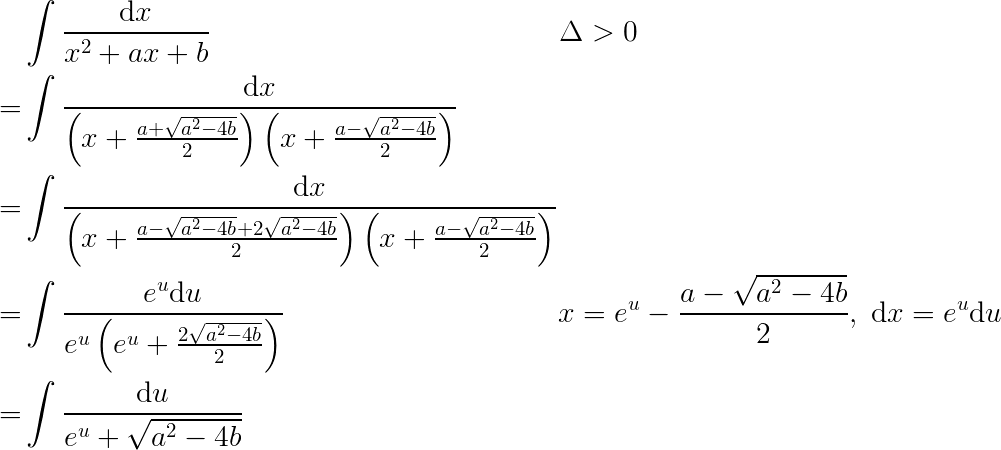It will become apparent why the determinant $\Delta$ cannot also be zero in further steps.

Now that I have contorted the integral to this particular form, I can use the same technique of conjuring an $e^x$ to appear in the numerator. I do this by first scaling up the numerator to match the constant in the denominator, then applying a $1-$ to the integral, by which time the integral can be solved with relative ease.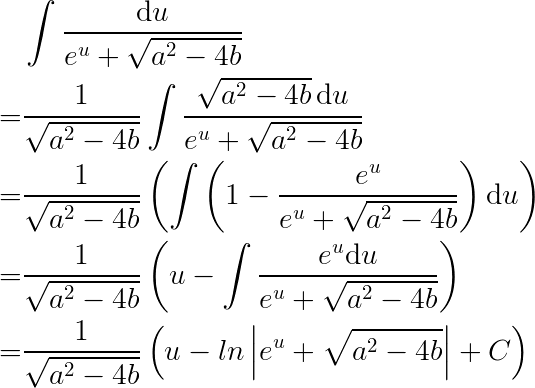Finally, I proceed to convert back to $x$ from $u$.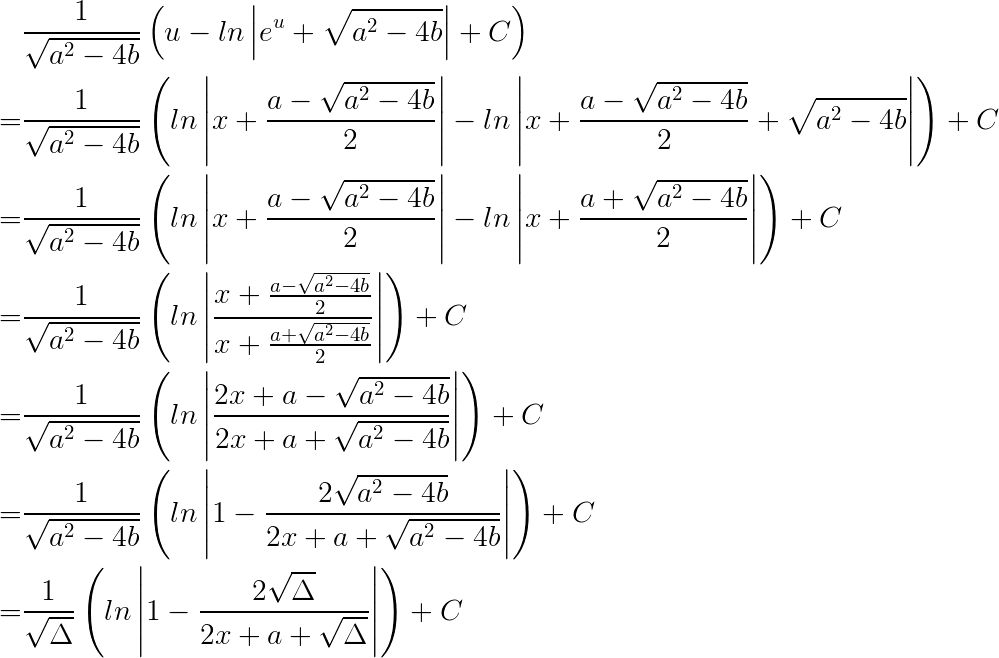Since we cannot divide by zero, the determinant cannot be zero.

To my amazement, this relation is actually on Wikipedia - another case where the determinant is negative is covered by Wolfram Alpha, and is also listed on Wikipedia.

But why stop here? I wanted to try to apply the same technique to rational functions where a constant divides a cubic or a higher power.

However, my efforts were in vain. The technique was only able to reduce one root of the quadratic by cancelling an $e^u$ term, which does not apply to higher powers where more roots exist. Moreover, it is difficult to find the roots to start with.

I started playing with random integrals that I could come up with via strange substitutions, such as: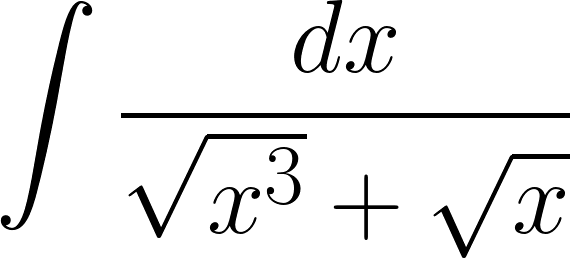I then came across this problem after I got really really bored: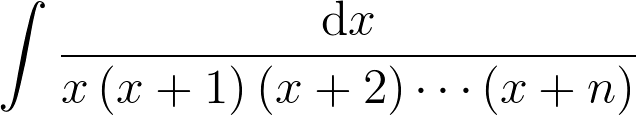where it was a final problem in a list of supposedly challenging integrals. And it really was challenging. And my previous method of substitution did no good whatsoever. However, I did eventually come up with a hideous looking solution: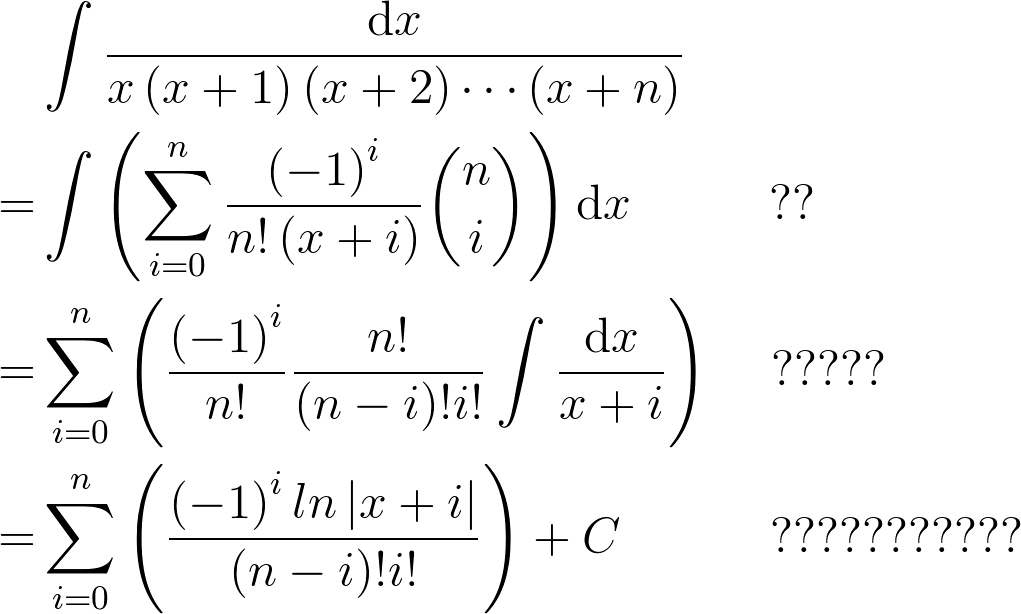with the increasing number of question marks denoting my disbelief in the accuracy of my work and notation and in the complexity of the non-existent actual solution.

Fruitful nonetheless.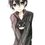Note by Lolly Lau
3 years ago

This discussion board is a place to discuss our Daily Challenges and the math and science related to those challenges. Explanations are more than just a solution — they should explain the steps and thinking strategies that you used to obtain the solution. Comments should further the discussion of math and science.

When posting on Brilliant:

• Use the emojis to react to an explanation, whether you're congratulating a job well done , or just really confused .
• Ask specific questions about the challenge or the steps in somebody's explanation. Well-posed questions can add a lot to the discussion, but posting "I don't understand!" doesn't help anyone.
• Try to contribute something new to the discussion, whether it is an extension, generalization or other idea related to the challenge.
• Stay on topic — we're all here to learn more about math and science, not to hear about your favorite get-rich-quick scheme or current world events.

MarkdownAppears as
*italics* or _italics_ italics
**bold** or __bold__ bold
- bulleted- list
• bulleted
• list
1. numbered2. list
1. numbered
2. list
Note: you must add a full line of space before and after lists for them to show up correctly
paragraph 1paragraph 2

paragraph 1

paragraph 2

[example link](https://brilliant.org)example link
> This is a quote
This is a quote
    # I indented these lines
# 4 spaces, and now they show
# up as a code block.

print "hello world"
# I indented these lines
# 4 spaces, and now they show
# up as a code block.

print "hello world"
MathAppears as
Remember to wrap math in $$ ... $$ or $ ... $ to ensure proper formatting.
2 \times 3 $2 \times 3$
2^{34} $2^{34}$
a_{i-1} $a_{i-1}$
\frac{2}{3} $\frac{2}{3}$
\sqrt{2} $\sqrt{2}$
\sum_{i=1}^3 $\sum_{i=1}^3$
\sin \theta $\sin \theta$
\boxed{123} $\boxed{123}$

## Comments

Sort by:

Top Newest

this is extendable to at least the cubic in the denominator for rational functions. I shall reveal an extended method when I have time.

- 2 years, 4 months ago

Log in to reply

×

Problem Loading...

Note Loading...

Set Loading...